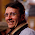## Sunday, May 8, 2011

### Find top log(n) or top sqt(n) values in an array of integers

Find top log(n) or top sqt(n) values in an array of integers in less than linear time.

1.I am lost here. Are we talking about worst-case single-threaded asymptotic time complexity?

I think it has to be at least O(n), because missing even a single entry in the array can alter the result.

What am I missing this time? :)

2.and if you need to find the top k?

3.If k=0, then it's easy - you just output an empty set.

If k>0, then the problem is at least as difficult as finding the 1 top element.

I am not sure if "top" means "most frequent" or "highest value", but in both cases you need to read all elements to be sure that your answer is right.

Example ("most frequent"): a small number of 1s, the same large number of 2s and 3s, and one unknown integer x.

Example ("highest value"): all elements equal to 10, except one unknown.